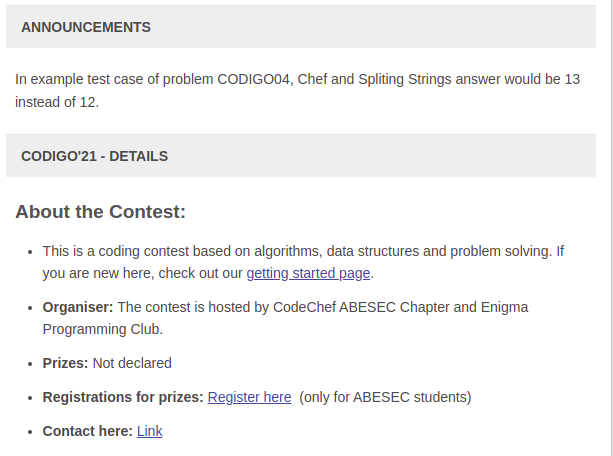# Help chef and split the string?

the problem looks easy but i am not getting idea to solve this one i have seen some of the submission but still not getting idea can anybody help with this?
problem : CodeChef: Practical coding for everyone

I think the sample Ouptut is invalid.

``````3
5 3
abcde
2 2
ok
3 1
yes
``````

For n = 5 and k = 3, the answer should be 13
Here are all possible ways of splitting abcde.

``````a-b-c-d-e
ab-c-d-e
a-bc-d-e
a-b-cd-e
a-b-c-de
ab-cd-e
a-bc-de
ab-c-de
abc-de
abc-d-e
a-bcd-e
ab-cde
a-b-cde
``````

The Complete Problem is trash. It is very disgusting to see the following code giving AC.

``````testcase=int(input())

while(testcase):
testcase=testcase-1
a,b=map(int,input().split())
string=input()
if(b==1):
print(1)
else:
print(a*b-b)
``````